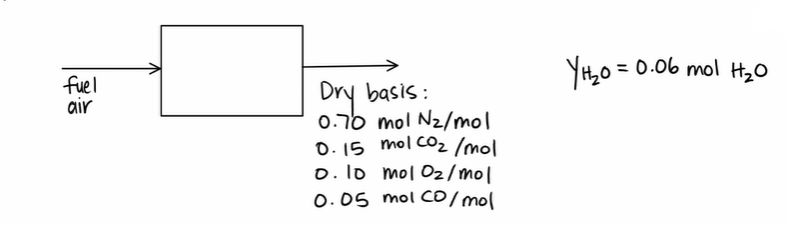LearnChemE

#### Wet and Dry Basis: Example Problems

Try to solve these problems before watching the solutions in the screencasts.

##### Example Problem 1

Convert a dry stack gas to a wet basis given the mole fraction of water in the product gas.##### Example Problem 2

When 20. mol/min of n-hexane and 500. mol/min of air are fed to a reactor, 50.% of the n-hexane oxidizes, and 90% of that is complete combustion. Determine the mole fractions of the product stream on a wet and a dry basis. Calculate the mole ratio of water to dry gas.